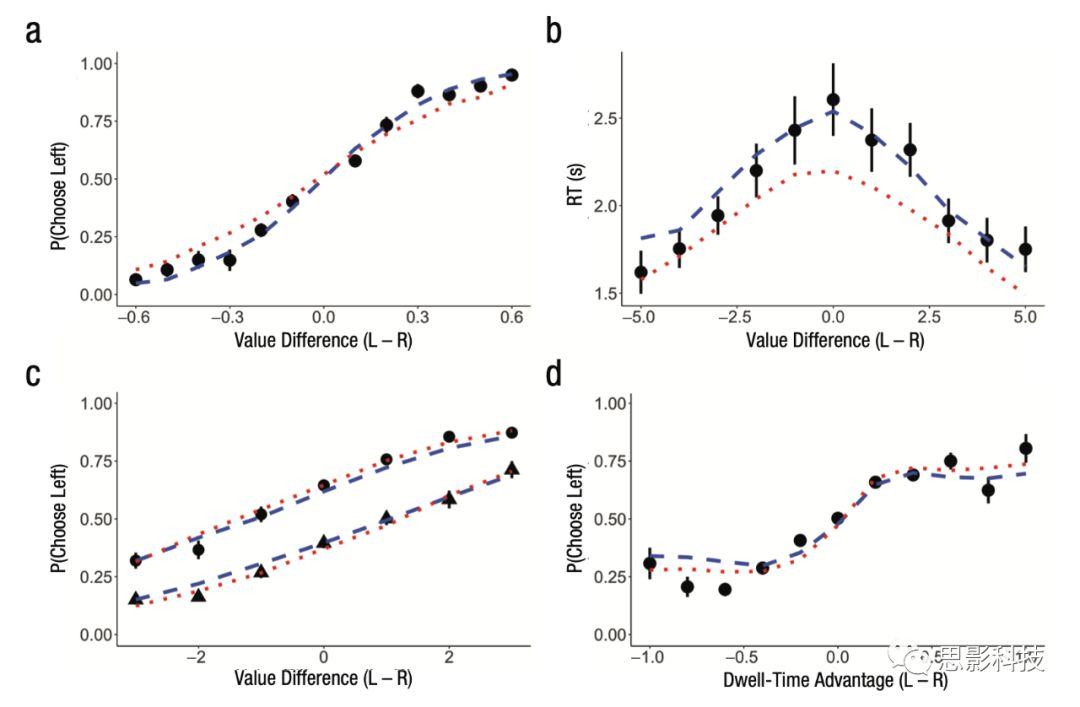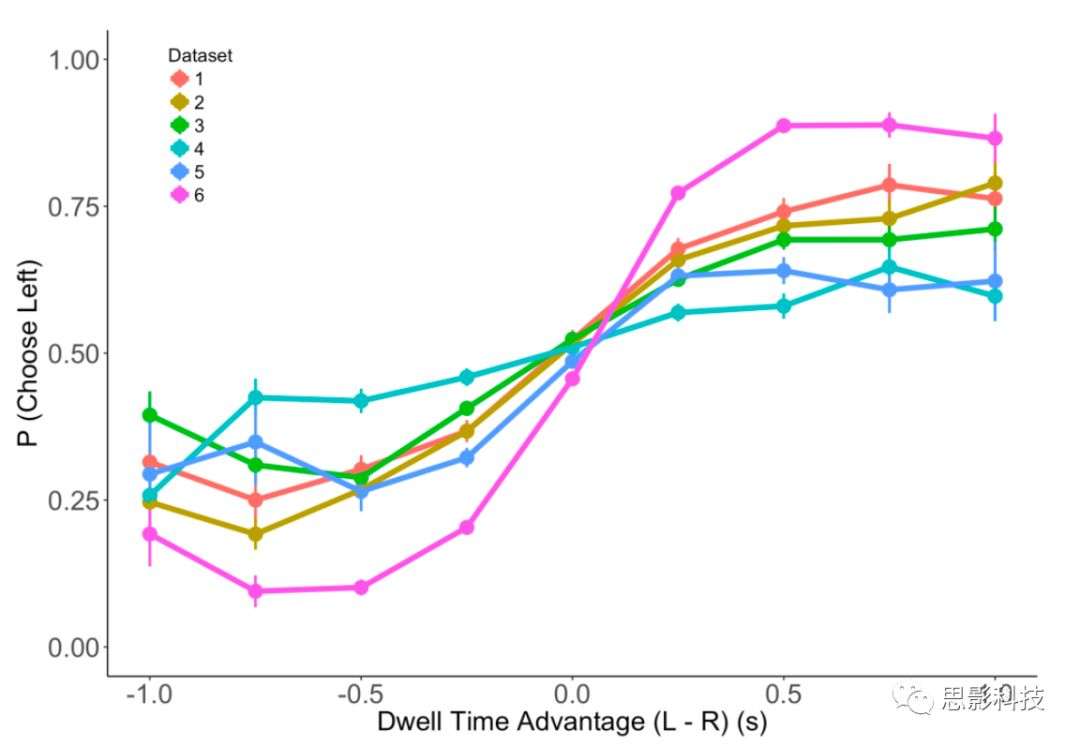# 眼动研究：决策注意中注视对物体价值的交互影响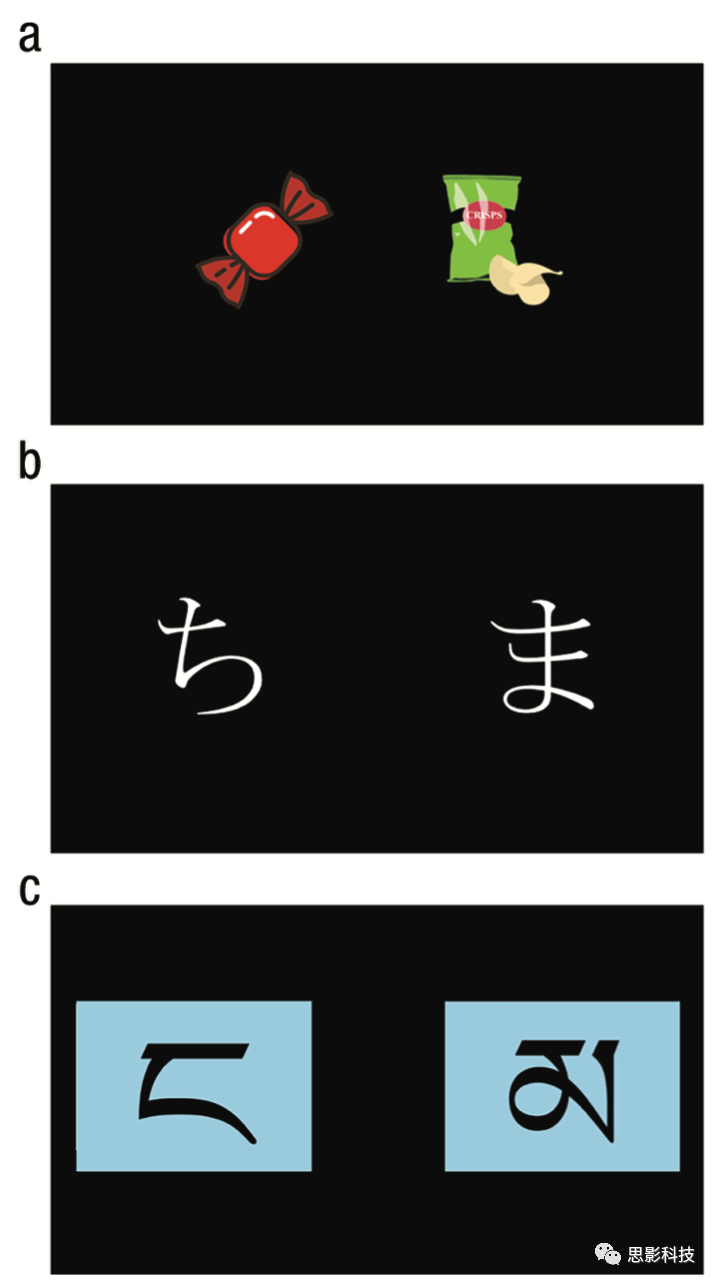Figure1Table 1

gaze left:ν= dUL-θUR

gaze right:ν= dθUL-UR

ULUR 是左右选项的主观价值，d是价值的尺度参数，θ是注意折扣参数。加法模型

gaze left:ν= dUL-UR+η

gaze right:ν= dUL-UR-η

η—捕获注意力效应参数。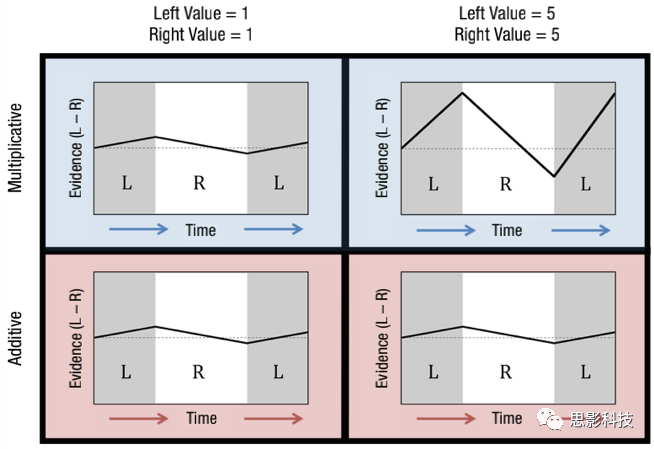Figure 2）乘法模型和加法模型的图示（上为乘法模型）

1）反应时（RT）与总体价值

log(RT)~β01|ULUR| + β2 (UL+UR).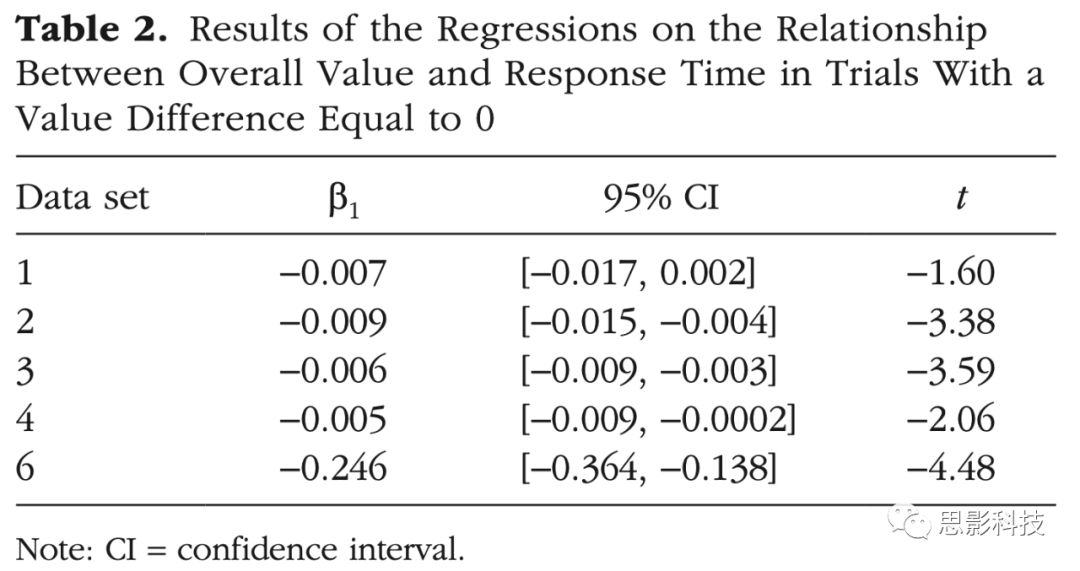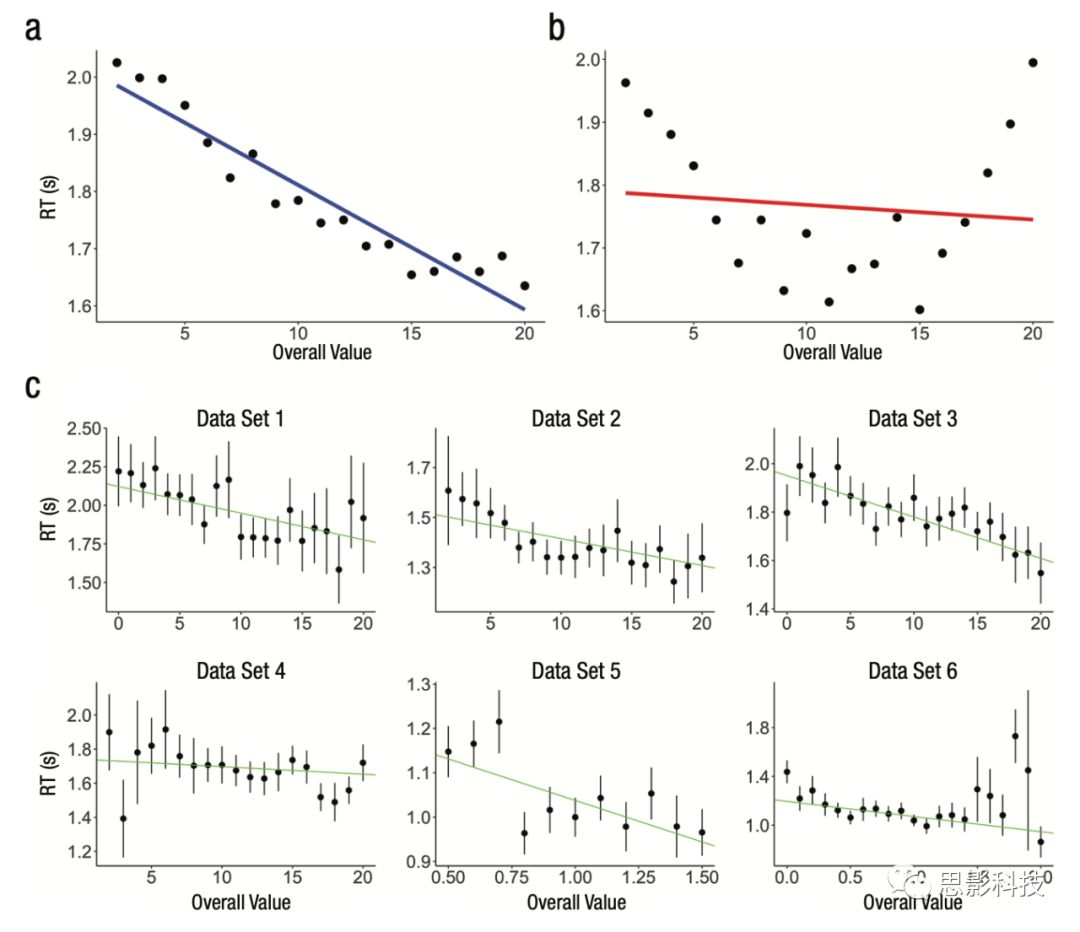Figure 3 加法模型和乘法模型在数据集中的测试

2）价值与注意力的交互建模

choose left ~ β0 + β1(UL UR) + β2 (UL + UR) + β3(left dwell proportion) |(left value < median (left value )) + β4 (left dwell proportion)|(left value ≥ median (left value)).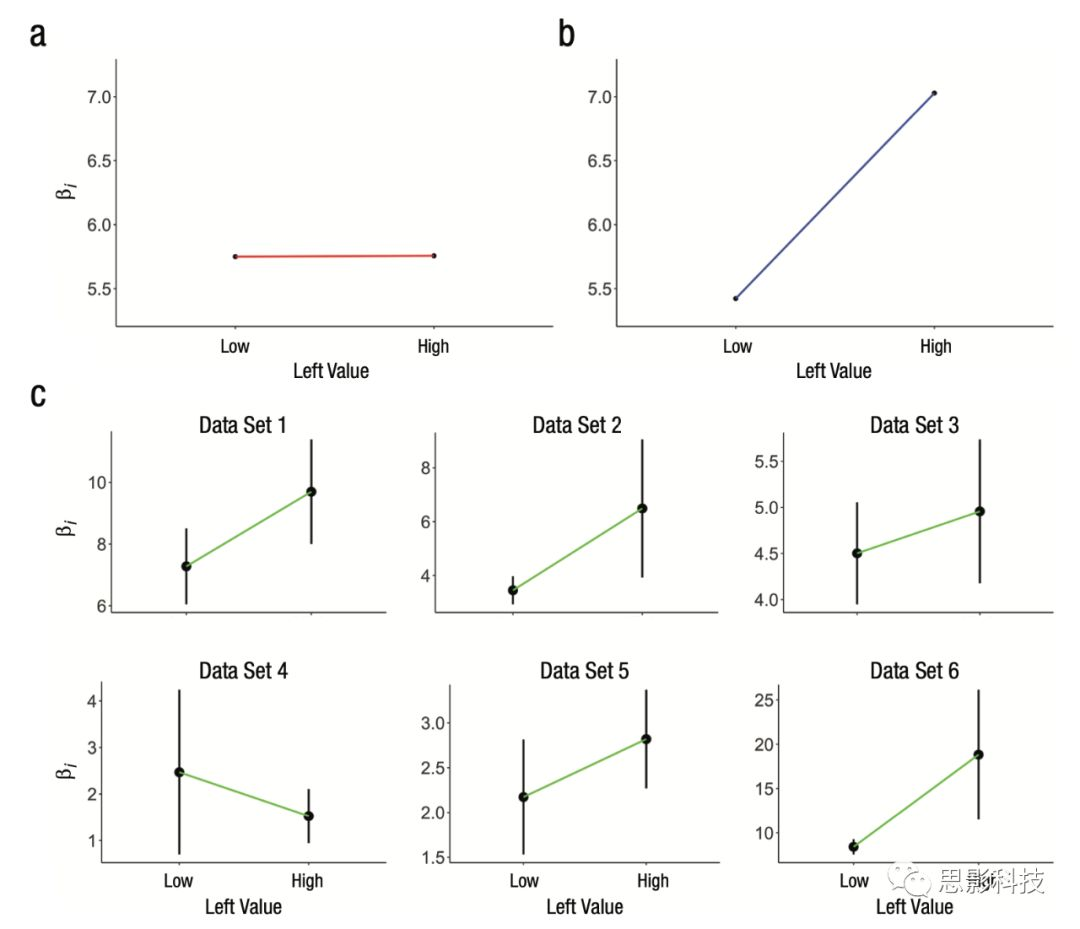Figure 4 交互模型中对β3β4参数的拟合

3）模型结果